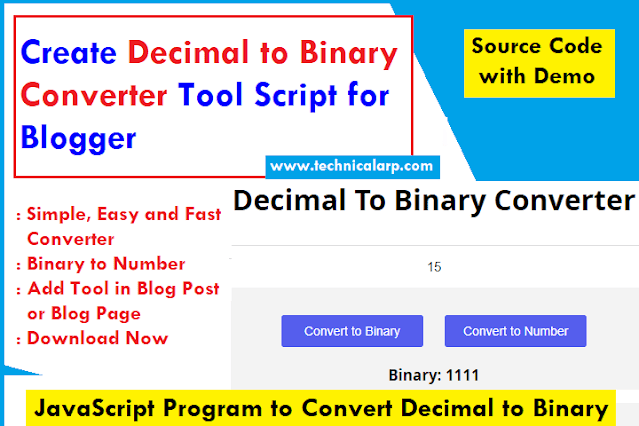# Decimal to Binary Converter Tool Script

Convert decimal number to binary number using our Decimal to Binary Converter tool. Yes! we can create our own decimal to binary converter tool website using HTML and JavaScript source code. In this article, I will be sharing the Decimal Number to Binary Converter Tool JavaScript code for bloggers or any platform.

Many students or any person want to convert decimal numbers into equivalent binary numbers then such a unique tool is used to convert Decimal numbers. And If you want to know convert decimal to binary in javascript code then stay with me.

An online tool to convert numbers into binary numbers is really helpful for a person like me, Why? because I am into the programming field. And as we know computers only understand binary numbers which are a combination of only 0 and 1.create decimal to binary converter tool script for blogger

You may also like our Random password generator tool script javascript source code

Now, if you don't know how it works and what it is? then let me try to tell you in a short summary.

## Decimal to Binary online converter tool

Our daily-used number is called a Decimal number which is the combination of 10 digits, including (0, 1, 2, 3, 4, 5, 6, 7, 8, 9).

And Computer only knows Zeros and One i.e Binary Number System.

In binary number has only 2 digits, including (0, 1). So basically number "7" in binary format will be "0111"

Well, in this article, We will not learn how logically it converts decimal numbers to binary numbers. Instead will create our own covert decimal to binary tool using JavaScript and HTML.

## How to Use the Decimal to Binary Converter Tool?

Convert decimal to the binary number or binary number to decimal number using our tool from below.

To use the number to binary converter:

• Enter any two or more than two numbers in the input box, for example, 7860.
• Click on the "Convert To Binary" Button.
• Now Convert Binary to Decimal Number paste or type binary number in the given text box.
• Click on the "Convert to Decimal" Button.
• That's it

## Online Decimal to Binary Converter

Fast & Simplest Decimal to Binary online converter tool. Just paste your decimal number & press the convert button to get a binary number.

### Decimal Number To Binary Converter Online Tool

For Quick reference below are some starting and most used decimal numbers converted to the equivalent binary values.

The table below shows decimal numbers and the equivalent binary number values.

Decimal numbers converted to the equivalent binary values
Decimal NumberBinary Number
00
11
210
311
4100
5101
6110
7111
81000
91001
101010
111011
121100
131101
141110
151111
1610000
1710001
1810010
1910011
2010100
2110101
2210110
2310111
2411000
2511001
2611010
2711011
2811100
2911101
3011110
3111111
32100000
641000000
12810000000
256100000000
5121000000000
102410000000000
2048100000000000

Now Do you want to make a Decimal Number to Binary Converter Tool in Blogger? or you can use JavaScript Program to Convert Decimal to Binary on any platform.

So let me provide our tool HTML, CSS, and JavaScript source code.

## Create Decimal to Binary Converter Tool in Blogger

To make your own number to binary converter tool website, you need to add HTML CSS and JavaScript (JQuery) codes in your website template/files. Just copy-paste from the below code and most import is converting decimal to binary in javascript code.

Want to increase website speed know about Minify CSS and JavaScript in Blogger

Steps to build Javascript-based Decimal to binary converter.

2. Copy HTML and paste it into your website where you want to show it.
3. Finally, add Convert Binary To Decimal Javascript source code.
4. Check it's working and then Congrats you have successfully created the tool script in blogger.

### Binary Converter Tool CSS code

``````<style type="text/css">
</style>
``````

### HTML for Converter tool

``````<div class="container" align="center">
<h1>Decimal To Binary Converter</h1> </div>
<div class="input-field">
<input type="text" id="input" autocomplete="off" placeholder="Enter a number" /> </div>
<div class="btn-result">
<div class="Btn">
<button id="n" class="click">Convert to Binary</button>
<button id='b'>Convert to Number</button>
</div>
</div>
</div>
``````

### JavaScript Program to Convert Decimal to Binary

Our JavaScript code is working fine as per our need, and you can modify it as per your need.

``````<script src='https://cdnjs.cloudflare.com/ajax/libs/jquery/2.1.3/jquery.min.js'></script>
<script>
function convert() {
var bi = document.getElementById('input').value;
var biArr = bi.split('');
biArr = biArr.reverse();
var final = [];
var pickle = [];
for(i = 0; i < biArr.length; i++) {
var banana = parseInt(biArr[i]);
pickle.push(banana);
var tomato = (Math.pow(2, i));
var potato = tomato * pickle[i];
final.push(potato);
}
var final2 = 0;
for(a = 0; a < final.length; a++) {
final2 = final2 + final[a];
}
document.getElementById('answer').innerHTML = "Number: " + final2;
}
convert()
})
var num;

function convert(num) {
var binary = [];
var num = document.getElementById("input").value;
num = parseInt(num);
if(!Number.isInteger(num)) {
return NaN;
}
while(num / 2 != 0) {
binary.unshift(num % 2);
num = Math.floor(num / 2);
}
if(binary.length == 2) {
binary.unshift("0");
}
if(binary.length == 3) {
binary.unshift("0");
}
if(binary.length == 1) {
binary.unshift("000");
}
binary = binary.join("");
return binary;
}
\$(".click").click(function() {
var insert = convert(num);
answer.innerHTML = "Binary: " + insert;
});
});
</script>
``````

Time for a conclusion.

Also, Converting Text to binary numbers is made easy using our Text to Binary Converter.

In Conclusion of Decimal to Binary Converter Tool Script

Are you there? Now, you know how to use this tool and also how to create a number to binary converter tool Right!.

So, I hope you found our tool and script we will come up with a more useful tool.

If you have any difficulty or want more such useful tools then please comment down.

See you in a new blog post.